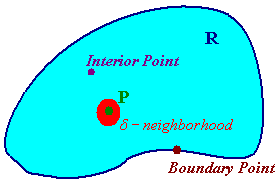$$\newcommand{\id}{\mathrm{id}}$$ $$\newcommand{\Span}{\mathrm{span}}$$ $$\newcommand{\kernel}{\mathrm{null}\,}$$ $$\newcommand{\range}{\mathrm{range}\,}$$ $$\newcommand{\RealPart}{\mathrm{Re}}$$ $$\newcommand{\ImaginaryPart}{\mathrm{Im}}$$ $$\newcommand{\Argument}{\mathrm{Arg}}$$ $$\newcommand{\norm}{\| #1 \|}$$ $$\newcommand{\inner}{\langle #1, #2 \rangle}$$ $$\newcommand{\Span}{\mathrm{span}}$$

# 1.3: 2D Limits

$$\newcommand{\vecs}{\overset { \rightharpoonup} {\mathbf{#1}} }$$ $$\newcommand{\vecd}{\overset{-\!-\!\rightharpoonup}{\vphantom{a}\smash {#1}}}$$$$\newcommand{\id}{\mathrm{id}}$$ $$\newcommand{\Span}{\mathrm{span}}$$ $$\newcommand{\kernel}{\mathrm{null}\,}$$ $$\newcommand{\range}{\mathrm{range}\,}$$ $$\newcommand{\RealPart}{\mathrm{Re}}$$ $$\newcommand{\ImaginaryPart}{\mathrm{Im}}$$ $$\newcommand{\Argument}{\mathrm{Arg}}$$ $$\newcommand{\norm}{\| #1 \|}$$ $$\newcommand{\inner}{\langle #1, #2 \rangle}$$ $$\newcommand{\Span}{\mathrm{span}}$$ $$\newcommand{\id}{\mathrm{id}}$$ $$\newcommand{\Span}{\mathrm{span}}$$ $$\newcommand{\kernel}{\mathrm{null}\,}$$ $$\newcommand{\range}{\mathrm{range}\,}$$ $$\newcommand{\RealPart}{\mathrm{Re}}$$ $$\newcommand{\ImaginaryPart}{\mathrm{Im}}$$ $$\newcommand{\Argument}{\mathrm{Arg}}$$ $$\newcommand{\norm}{\| #1 \|}$$ $$\newcommand{\inner}{\langle #1, #2 \rangle}$$ $$\newcommand{\Span}{\mathrm{span}}$$

## Topology Terminology

Let $$P$$ be a point in the plane then a d-neighborhood (ball) of $$P$$ is the set of points that are less than $$d$$ units away from $$P$$. If $$R$$ is a region, then a point $$P$$ is called an interior point of $$R$$ if there is a d-neighborhood totally contained in $$R$$. If every point of $$R$$ is an interior point of $$R$$ then $$R$$ is called open. A point $$P$$ is called a boundary point of $$R$$ if every d-neighborhood of $$R$$ contains both points in $$R$$ and not in $$R$$. $$R$$ is called closed if it contains all of its boundary points.Definition: Two-Dimensional Limits

Let $$f(x,y)$$ be a function defined near the point $$P$$, then

$\lim_{x \rightarrow P} f(x,y)= L$

if there is a $$d$$ such that $$f(x,y)$$ is close to $$L$$ for all points (except possibly $$P$$) in the d - neighborhood of $$P$$.

Equivalently, the limit is $$L$$ if for all paths that lead to $$P$$, the function also tends towards $$P$$. (Recall that for the one variable case we needed to check only the path from the left and from the right.) To show that a limit does not exist at a point, it is necessary to demonstration that two paths that both lead to $$P$$ such that $$f(x,y)$$ tends towards different values.

## Techniques For Finding Limits

Example $$\PageIndex{1}$$

Show that

$\lim_{(x,y) \rightarrow (0,0)} \dfrac{xy}{x^2+y^2}$

does not exist.

Solution

First select the path along the x-axis. On this path

$y = 0$

so the function becomes:

$f(x,0)=\dfrac{0}{x^2} = 0.$

Now choose the path along the y = x line:

$f(0,y)= \dfrac{x^2}{2x^2} = \dfrac{1}{2}.$

Hence the function tends towards two different values for different paths. We can conclude that the limit does not exist. The graph is pictured below.Example $$\PageIndex{2}$$

Find

$\lim_{(x,y) \rightarrow (0,0)} \dfrac{x^3+y^3}{x^2+y^2}.$

Solution

We could try the paths from the last example, but both paths give a value of 0 for the limit. Hence we suspect that the limit exists. We convert to polar coordinates and take the limit as $$r$$ approaches 0:

We have

$f(r,q) =\dfrac{r^3\cos^3q + r^3\sin^3q}{ r^2} = r\cos^3q + r\sin^3q$

as $$r$$ approaches 0, the function also approaches 0 no matter what $$q$$ is. Hence the limit is 0. Below is the graph of this function.Exercises

Find the limit if it exists

1. $$\lim_{(x,y) \rightarrow (0,1)} \dfrac{xy-x}{x^2+y^2-2y+1}$$
2. $$\lim_{(x,y) \rightarrow (0,1)} \dfrac{4x^2y^2}{x^4+y^4}$$

## Continuity

We make the following definition for continuity.

Definition: Continuity

A function of several variables is continuous at a point $$P$$ if the limit exists at $$P$$ and the function defined at $$P$$ is equal to this limit.

As with functions of one variable, polynomials are continuous, sums, products, and compositions of continuous functions are continuous. Quotients of continuous functions are continuous. A function is continuous if it is continuous at every point.

Larry Green (Lake Tahoe Community College)

• Integrated by Justin Marshall.